Courses

# Extra MCQ For Chapter 13 - Symmetry, Class 6, Math

## 20 Questions MCQ Test | Extra MCQ For Chapter 13 - Symmetry, Class 6, Math

Description
This mock test of Extra MCQ For Chapter 13 - Symmetry, Class 6, Math for Class 6 helps you for every Class 6 entrance exam. This contains 20 Multiple Choice Questions for Class 6 Extra MCQ For Chapter 13 - Symmetry, Class 6, Math (mcq) to study with solutions a complete question bank. The solved questions answers in this Extra MCQ For Chapter 13 - Symmetry, Class 6, Math quiz give you a good mix of easy questions and tough questions. Class 6 students definitely take this Extra MCQ For Chapter 13 - Symmetry, Class 6, Math exercise for a better result in the exam. You can find other Extra MCQ For Chapter 13 - Symmetry, Class 6, Math extra questions, long questions & short questions for Class 6 on EduRev as well by searching above.
QUESTION: 1

Solution:
QUESTION: 2

Solution:
QUESTION: 3

### How many lines of symmetry does a rectangle have?

Solution:
QUESTION: 4

How many lines of symmetry does a circle have?

Solution:
QUESTION: 5

Find the vertical mirror image of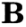Solution:
QUESTION: 6

Which of the following letters of the English alphabet has a vertical line of symmetry?

Solution:
QUESTION: 7

Find the number of lines of symmetry for the following shape.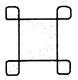Solution: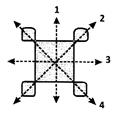QUESTION: 8

In a Δ AB,AB = AC and AD⊥BC,BE⊥AC and CF ⊥ AB. Then about which of the following is the triangle symmetrical?

Solution: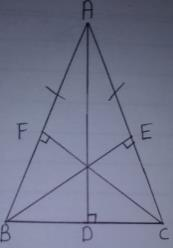⇒  It is given that in △ABC, AB = AC

∴ △ABC is an Isosceles triangle.

⇒  And in isosceles triangle line of symmetry is one.

QUESTION: 9

ABCD is a kite in which AB = AD and BC = DC. Which of the following is the kite symmetrical about?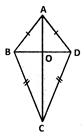Solution:
QUESTION: 10

How many lines of symmetry does the letter Z of the English alphabet have?

Solution:
QUESTION: 11

Solution:
QUESTION: 12

Determine the number of lines of symmetry for the following shape.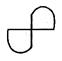Solution:

The given figure does not have any line of symmetry.

QUESTION: 13

Find the number of lines of symmetry of the figure given.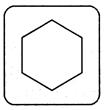Solution:

The figure is a regular hexagon.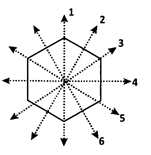QUESTION: 14

Which of the following has 5 lines of symmetry?

Solution: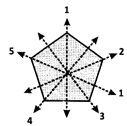A regular pentagon has 5 equal sides. So the line passing through each vertex to the each opposite side will be a line of symmetry.

QUESTION: 15

Identify the figure that completes the given figure, such that l is the line of symmetry.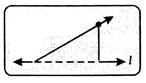Solution:

The symmetrical part of the given figure about the line l is the mirror image of the given figure.

QUESTION: 16

What is the number of lines of symmetry for the following shape?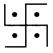Solution:

The given figure has no line symmetry.

QUESTION: 17

Which of the following figures are symmetrical with respect to exactly two lines?

Solution:
QUESTION: 18

How many lines of symmetry does the given figure have?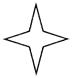Solution:
QUESTION: 19

Identify the number of lines of symmetry for the figure given.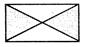Solution:
QUESTION: 20

Identify the number of lines of symmetry of the following figure.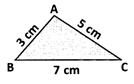Solution: